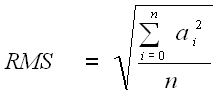﻿ Root Mean Square
Nevron .NET Vision Documentation
Root Mean Square

Syntax

The Root Mean Square is implemented as a composite function. The expression is:

POW(AVERAGE(POW(arg1; 2)); 0.5)
Arguments
The 'arg1' argument must be an array.
Result
The result is a scalar value.
Description

The formula of the Root Mean Square is:Where 'a' is an array of elements and n is the number of the elements.

Related Examples
Windows Forms: All Examples\Data Manipulation\Functions\Statistical\Count, Standard Deviation, RMS